# stem3

Plot 3-D discrete sequence data

## Syntax

``stem3(Z)``
``stem3(X,Y,Z)``
``stem3(___,'filled')``
``stem3(___,LineSpec)``
``stem3(___,Name,Value)``
``stem3(ax,___)``
``h = stem3(___)``

## Description

example

````stem3(Z)` plots entries in `Z` as stems extending from the xy-plane and terminating with circles at the entry values. The stem locations in the xy-plane are automatically generated. ```

example

````stem3(X,Y,Z)` plots entries in `Z` as stems extending from the xy-plane where `X` and `Y` specify the stem locations in the xy-plane. The inputs `X`, `Y`, and `Z` must be vectors or matrices of the same size. ```

example

````stem3(___,'filled')` fills the circles. Use this option with any of the input argument combinations in the previous syntaxes.```

example

````stem3(___,LineSpec)` specifies the line style, marker symbol, and color.```

example

````stem3(___,Name,Value)` modifies the stem chart using one or more name-value pair arguments. ```

example

````stem3(ax,___)` plots into the axes specified by `ax` instead of into the current axes (`gca`). The option, `ax`, can precede any of the input argument combinations in the previous syntaxes.```

example

````h = stem3(___)` returns the `Stem` object `h`. ```

## Examples

collapse all

Create a 3-D stem plot of cosine values between $-\pi /2$ and $\pi /2$ with a row vector input.

```figure X = linspace(-pi/2,pi/2,40); Z = cos(X); stem3(Z)```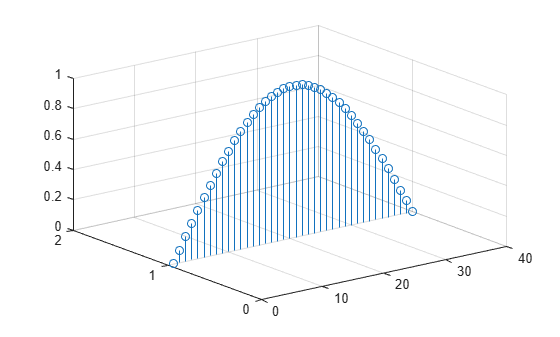`stem3` plots elements of `Z` against the same y value at equally space x values.

Create a 3-D stem plot of cosine values between $-\pi /2$ and $\pi /2$ with a column vector input.

```figure X = linspace(-pi/2,pi/2,40)'; Z = cos(X); stem3(Z)````stem3` plots elements of `Z` against the same x value at equally space y values.

Create a 3-D stem plot of sine and cosine values between $-\pi /2$ and $\pi /2$ with a matrix input.

```figure X = linspace(-pi/2,pi/2,40); Z = [sin(X); cos(X)]; stem3(Z)```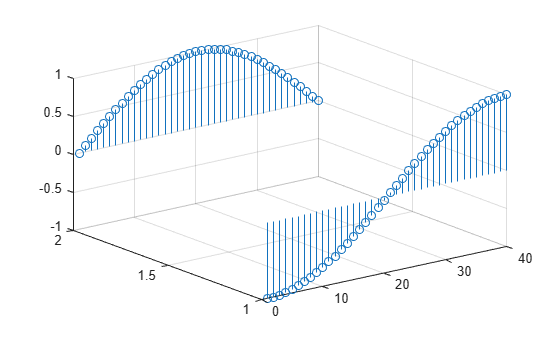`stem3` plots each row of `Z` against the same y value at equally space x values.

Create a 3-D stem plot and specify the stem locations along a curve. Use `view` to adjust the angle of the axes in the figure.

```figure X = linspace(-5,5,60); Y = cos(X); Z = X.^2; stem3(X,Y,Z) view(-8,30)```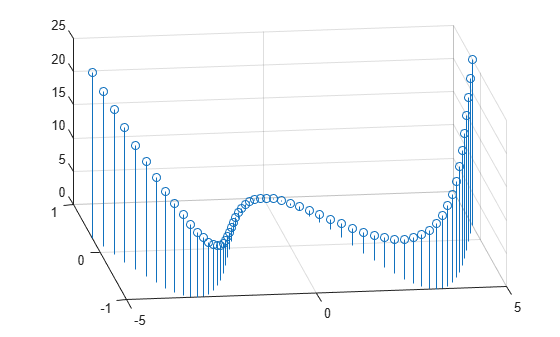`X` and `Y` determine the stem locations. `Z` determines the marker heights.

Create a 3-D stem plot with matrix data and specify the stem locations in the xy-plane.

```figure [X,Y] = meshgrid(0:.1:1); Z = exp(X+Y); stem3(X,Y,Z)````X` and `Y` determine the stem locations. `Z` determines the marker heights.

Create a 3-D stem plot of cosine values between $-\pi$ and $\pi$ and fill in the markers.

```X = linspace(-pi,pi,40); Z = cos(X); stem3(Z,'filled')```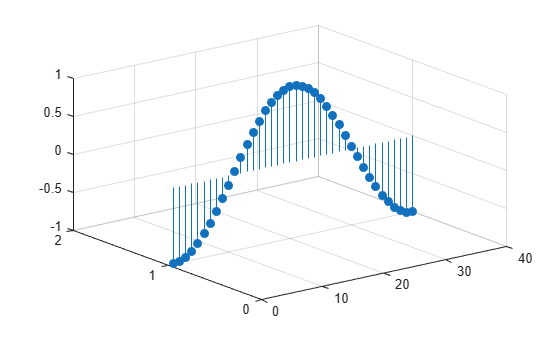Create a 3-D stem plot of cosine values between $-\pi$ and $\pi$. Use a dashed line style for the stem, set the marker symbols to stars, and set the color to magenta.

```figure X = linspace(-pi,pi,40); Z = cos(X); stem3(Z,'--*m')```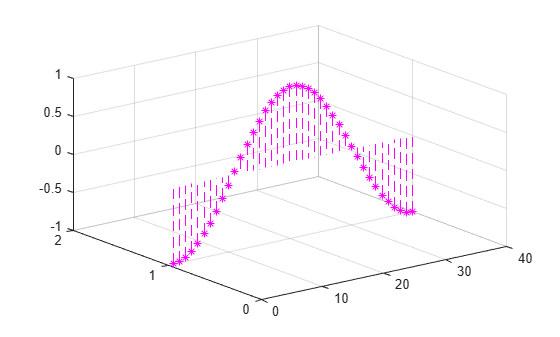To specify only two of the three `LineSpec` options, omit the third option from the character vector. For example, `'*m'` sets the marker symbol and the color and uses the default line style.

Create a 3-D stem plot and specify the stem locations along a circle. Set the stem to a dotted line style, the marker symbols to stars, and the color to magenta.

```figure theta = linspace(0,2*pi); X = cos(theta); Y = sin(theta); Z = theta; stem3(X,Y,Z,':*m')````X` and `Y` determine the stem locations. `Z` determines the marker heights.

Create a 3-D stem plot of cosine values between $-\pi$ and $\pi$. Set the marker symbols to squares with green faces and magenta edges.

```figure X = linspace(-pi,pi,40); Z = cos(X); stem3(Z,'Marker','s',... 'MarkerEdgeColor','m',... 'MarkerFaceColor','g')```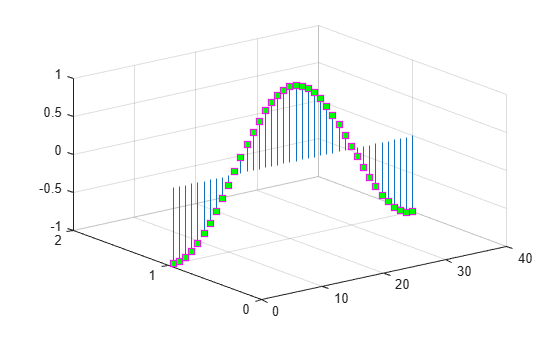Starting in R2019b, you can display a tiling of plots using the `tiledlayout` and `nexttile` functions. Call the `tiledlayout` function to create a 2-by-1 tiled chart layout. Call the `nexttile` function to create the axes objects `ax1` and `ax2`. Create separate stem plots in the axes by specifying the axes object as the first argument to `stem3`.

```X = linspace(-2,2,50); Y = X.^3; Z = exp(X); tiledlayout(2,1) % Top plot ax1 = nexttile; stem(ax1,X,Z) % Bottom plot ax2 = nexttile; stem3(ax2,X,Y,Z)```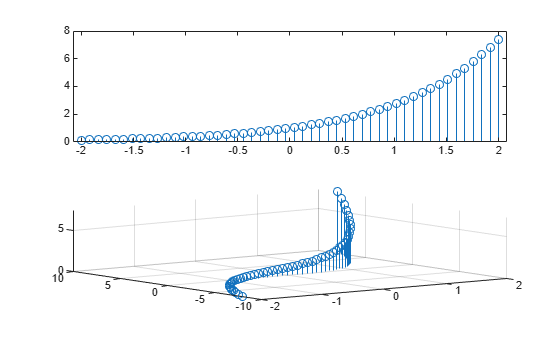Create a 3-D stem plot and return the stem series object.

```X = linspace(0,2); Y = X.^3; Z = exp(X).*cos(Y); h = stem3(X,Y,Z,'filled');```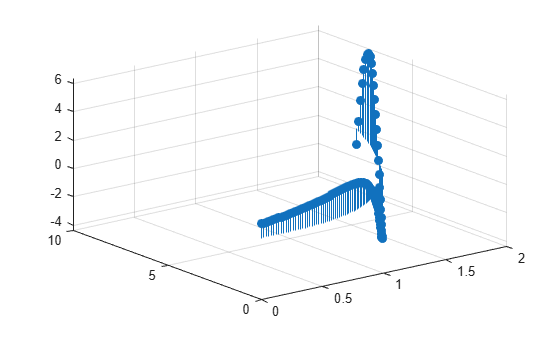Change the color to magenta and set the marker face color to yellow. Use `view` to adjust the angle of the axes in the figure. Use dot notation to set properties.

```h.Color = 'm'; h.MarkerFaceColor = 'y'; view(-10,35)```## Input Arguments

collapse all

Data sequence to display, specified as a vector or matrix. `stem3` plots each element in `Z` as a stem extending from the xy-plane and terminating at the data value.

• If `Z` is a row vector, `stem3` plots all elements against the same y value at equally spaced x values.

• If `Z` is a column vector, `stem3` plots all elements against the same x value at equally spaced y values.

• If `Z` is a matrix, `stem3` plots each row of `Z` against the same y value at equally spaced x values.

Data Types: `single` | `double` | `int8` | `int16` | `int32` | `int64` | `uint8` | `uint16` | `uint32` | `uint64` | `logical`

Locations to plot values of`Z`, specified as a vector or a matrix. Inputs `X`, `Y` and `Z` must be vectors or matrices of the same size.

Data Types: `single` | `double` | `int8` | `int16` | `int32` | `int64` | `uint8` | `uint16` | `uint32` | `uint64` | `logical`

Locations to plot values of `Z`, specified as a vector or a matrix. Inputs `X`, `Y` and `Z` must be vectors or matrices of the same size.

Data Types: `single` | `double` | `int8` | `int16` | `int32` | `int64` | `uint8` | `uint16` | `uint32` | `uint64` | `logical`

Line style, marker, and color, specified as a character vector or string containing symbols. The symbols can appear in any order. You do not need to specify all three characteristics (line style, marker, and color). For example, if you omit the line style and specify the marker, then the plot shows only the marker and no line.

Example: `'--or'` is a red dashed line with circle markers

Line StyleDescription
`-`Solid line
`--`Dashed line
`:`Dotted line
`-.`Dash-dot line
MarkerDescription
`'o'`Circle
`'+'`Plus sign
`'*'`Asterisk
`'.'`Point
`'x'`Cross
`'_'`Horizontal line
`'|'`Vertical line
`'s'`Square
`'d'`Diamond
`'^'`Upward-pointing triangle
`'v'`Downward-pointing triangle
`'>'`Right-pointing triangle
`'<'`Left-pointing triangle
`'p'`Pentagram
`'h'`Hexagram
ColorDescription

`y`

yellow

`m`

magenta

`c`

cyan

`r`

red

`g`

green

`b`

blue

`w`

white

`k`

black

`Axes` object. If you do not specify the axes, then `stem` plots into the current axes.

### Name-Value Pair Arguments

Specify optional comma-separated pairs of `Name,Value` arguments. `Name` is the argument name and `Value` is the corresponding value. `Name` must appear inside quotes. You can specify several name and value pair arguments in any order as `Name1,Value1,...,NameN,ValueN`.

Example: `'LineStyle',':','MarkerFaceColor','red'` plots the stem as a dotted line and sets the marker face color to red.

The properties listed here are only a subset. For a complete list, see Stem Properties.

Line style, specified as one of the options listed in this table.

Line StyleDescriptionResulting Line
`'-'`Solid line`'--'`Dashed line`':'`Dotted line`'-.'`Dash-dotted line`'none'`No lineNo line

Line width of stem and marker edge, specified as a positive value in point units.

Example: `0.75`

Stem color, specified as an RGB triplet, a hexadecimal color code, a color name, or a short name.

For a custom color, specify an RGB triplet or a hexadecimal color code.

• An RGB triplet is a three-element row vector whose elements specify the intensities of the red, green, and blue components of the color. The intensities must be in the range `[0,1]`; for example, ```[0.4 0.6 0.7]```.

• A hexadecimal color code is a character vector or a string scalar that starts with a hash symbol (`#`) followed by three or six hexadecimal digits, which can range from `0` to `F`. The values are not case sensitive. Thus, the color codes `'#FF8800'`, `'#ff8800'`, `'#F80'`, and `'#f80'` are equivalent.

Alternatively, you can specify some common colors by name. This table lists the named color options, the equivalent RGB triplets, and hexadecimal color codes.

Color NameShort NameRGB TripletHexadecimal Color CodeAppearance
`'red'``'r'``[1 0 0]``'#FF0000'``'green'``'g'``[0 1 0]``'#00FF00'``'blue'``'b'``[0 0 1]``'#0000FF'``'cyan'` `'c'``[0 1 1]``'#00FFFF'``'magenta'``'m'``[1 0 1]``'#FF00FF'``'yellow'``'y'``[1 1 0]``'#FFFF00'``'black'``'k'``[0 0 0]``'#000000'``'white'``'w'``[1 1 1]``'#FFFFFF'``'none'`Not applicableNot applicableNot applicableNo color

Here are the RGB triplets and hexadecimal color codes for the default colors MATLAB® uses in many types of plots.

`[0 0.4470 0.7410]``'#0072BD'``[0.8500 0.3250 0.0980]``'#D95319'``[0.9290 0.6940 0.1250]``'#EDB120'``[0.4940 0.1840 0.5560]``'#7E2F8E'``[0.4660 0.6740 0.1880]``'#77AC30'``[0.3010 0.7450 0.9330]``'#4DBEEE'``[0.6350 0.0780 0.1840]``'#A2142F'`Example: `'blue'`

Example: ```[0 0 1]```

Example: `'#0000FF'`

Marker symbol, specified as one of the markers listed in this table.

ValueDescription
`'o'`Circle
`'+'`Plus sign
`'*'`Asterisk
`'.'`Point
`'x'`Cross
`'_'`Horizontal line
`'|'`Vertical line
`'square'` or `'s'`Square
`'diamond'` or `'d'`Diamond
`'^'`Upward-pointing triangle
`'v'`Downward-pointing triangle
`'>'`Right-pointing triangle
`'<'`Left-pointing triangle
`'pentagram'` or `'p'`Five-pointed star (pentagram)
`'hexagram'` or `'h'`Six-pointed star (hexagram)
`'none'`No markers

Example: `'+'`

Example: `'diamond'`

Marker size, specified as a positive value in points, where 1 point = 1/72 of an inch.

Marker outline color, specified as `'auto'`, an RGB triplet, a hexadecimal color code, a color name, or a short name. The default value of `'auto'` uses the same color as the `Color` property.

For a custom color, specify an RGB triplet or a hexadecimal color code.

• An RGB triplet is a three-element row vector whose elements specify the intensities of the red, green, and blue components of the color. The intensities must be in the range `[0,1]`; for example, ```[0.4 0.6 0.7]```.

• A hexadecimal color code is a character vector or a string scalar that starts with a hash symbol (`#`) followed by three or six hexadecimal digits, which can range from `0` to `F`. The values are not case sensitive. Thus, the color codes `'#FF8800'`, `'#ff8800'`, `'#F80'`, and `'#f80'` are equivalent.

Alternatively, you can specify some common colors by name. This table lists the named color options, the equivalent RGB triplets, and hexadecimal color codes.

Color NameShort NameRGB TripletHexadecimal Color CodeAppearance
`'red'``'r'``[1 0 0]``'#FF0000'``'green'``'g'``[0 1 0]``'#00FF00'``'blue'``'b'``[0 0 1]``'#0000FF'``'cyan'` `'c'``[0 1 1]``'#00FFFF'``'magenta'``'m'``[1 0 1]``'#FF00FF'``'yellow'``'y'``[1 1 0]``'#FFFF00'``'black'``'k'``[0 0 0]``'#000000'``'white'``'w'``[1 1 1]``'#FFFFFF'``'none'`Not applicableNot applicableNot applicableNo color

Here are the RGB triplets and hexadecimal color codes for the default colors MATLAB uses in many types of plots.

`[0 0.4470 0.7410]``'#0072BD'``[0.8500 0.3250 0.0980]``'#D95319'``[0.9290 0.6940 0.1250]``'#EDB120'``[0.4940 0.1840 0.5560]``'#7E2F8E'``[0.4660 0.6740 0.1880]``'#77AC30'``[0.3010 0.7450 0.9330]``'#4DBEEE'``[0.6350 0.0780 0.1840]``'#A2142F'`Marker fill color, specified as `'auto'`, an RGB triplet, a hexadecimal color code, a color name, or a short name. The `'auto'` option uses the same color as the `Color` property of the parent axes. If you specify `'auto'` and the axes plot box is invisible, the marker fill color is the color of the figure.

For a custom color, specify an RGB triplet or a hexadecimal color code.

• An RGB triplet is a three-element row vector whose elements specify the intensities of the red, green, and blue components of the color. The intensities must be in the range `[0,1]`; for example, ```[0.4 0.6 0.7]```.

• A hexadecimal color code is a character vector or a string scalar that starts with a hash symbol (`#`) followed by three or six hexadecimal digits, which can range from `0` to `F`. The values are not case sensitive. Thus, the color codes `'#FF8800'`, `'#ff8800'`, `'#F80'`, and `'#f80'` are equivalent.

Alternatively, you can specify some common colors by name. This table lists the named color options, the equivalent RGB triplets, and hexadecimal color codes.

Color NameShort NameRGB TripletHexadecimal Color CodeAppearance
`'red'``'r'``[1 0 0]``'#FF0000'``'green'``'g'``[0 1 0]``'#00FF00'``'blue'``'b'``[0 0 1]``'#0000FF'``'cyan'` `'c'``[0 1 1]``'#00FFFF'``'magenta'``'m'``[1 0 1]``'#FF00FF'``'yellow'``'y'``[1 1 0]``'#FFFF00'``'black'``'k'``[0 0 0]``'#000000'``'white'``'w'``[1 1 1]``'#FFFFFF'``'none'`Not applicableNot applicableNot applicableNo color

Here are the RGB triplets and hexadecimal color codes for the default colors MATLAB uses in many types of plots.

`[0 0.4470 0.7410]``'#0072BD'``[0.8500 0.3250 0.0980]``'#D95319'``[0.9290 0.6940 0.1250]``'#EDB120'``[0.4940 0.1840 0.5560]``'#7E2F8E'``[0.4660 0.6740 0.1880]``'#77AC30'``[0.3010 0.7450 0.9330]``'#4DBEEE'``[0.6350 0.0780 0.1840]``'#A2142F'`## Output Arguments

collapse all

`Stem` object. This is a unique identifier, which you can use to modify the properties of the `Stem` object after it is created.

## Extended Capabilities

### Topics

Introduced before R2006a